EXERCISE 29 — RISING/SETTING AZIMUTH -SUN (Numerical Solution) |

# EXERCISE 29 — RISING/SETTING AZIMUTH -SUN (Numerical Solution)

1. ###### On 2nd Sept 2008, in DR 40˚ 28’N 064˚ 20’E, the rising sun bore 090˚(C). If variation was 5˚ W, find the deviation of the compass.

LMT Sunrise         = 2d  05h  28m  00s
LIT (E)                  = (-)   04h  17m   20s
GMT Sunrise      =  2d   01h   10m  40s
Dec (sun ) 2d 01h  10m  40s
=  07˚  50.3’  N

As we know that:
LMT of Sunrise or Sunset are taken from the nautical almanac and needs only be interpolated and taken in whole minutes of time. If the time ofobservation is given in the problem, then given time should be used for calculation. It is necessary to remove the ambiquity of chron time.

We know that: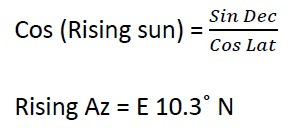###### NOTE:

NAMING OF AMPLITUDE: The prefix of True Amplitude is E if the body is rising and W if the body is setting. The suffix is same as that of the declination.

T Az          = 079.7˚ (T)
C Az          = 090.0˚  (C)
Error         = 10.3˚ W
Variation  = 05˚ W
Deviation = 5.3 W

1. ###### On 1st May 2008, in DR 30˚ 06’N 179 ˚ 45’W, the setting sun bore 285˚(C). If variation was 2˚W, find the deviation for the ship’s head.

LMT Sunrise    = 1d   18h    38m  00s
LIT (W)             = (+)   11h    59m  00s
GMT Sunset    = 2d    06h   37m  00s

As we know that:
LMT of Sunrise or Sunset are taken from the nautical almanac and needs only be interpolated and taken in whole minutes of time. If the time ofobservation is given in the problem, then given time should be used for calculation. It is necessary to remove the ambiquity of chron time.

Dec sun  2d  06h                = N 15˚  30.1’
d (+0.7)                                =    +       00.4’
Dec 2d  06h  37m  00s      = N 15˚  30.5’

We know that :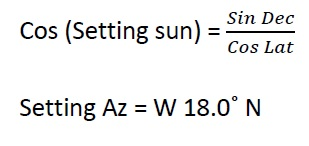NOTE:

NAMING OF AMPLITUDE: The prefix of True Amplitude is E if the body is rising and W if the body is setting. The suffix is same as that of the declination.

T Az          = 288.0˚ (T)
C Az          = 285.0˚  (C)
Error          = 03.0˚ E
Variation   = 02˚ W
Deviation  = 5.0˚E

1. ###### Jan 20th 2008, in DR 54˚ 20’S 046 ˚ 27’W, the sun set bearing 234˚(C). If variation was 3˚ W, find the deviation of the compass.

LMT Sunrise       = 20d   20h  21m  00s
LIT (W)                   = (+) 03h  05m  48s
GMT Sunset        = 20d   23h  26m  48s

Dec sun  20d  06h            = S 20˚  05.7’
d (-0.5)                               =       –   00.2’
Dec 2d  06h  37m  00s     = S20˚  05.5’

We know that :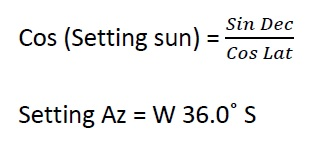NOTE:

NAMING OF AMPLITUDE: The prefix of True Amplitude is E if the body is rising and W if the body is setting. The suffix is same as that of the declination.

T Az          = 234.0˚ (T)
C Az          = 234.0˚  (C)
Error         = NIL
Variation  = 03˚ W
Deviation = 3.0 W

1. ###### Nov 30th 2008, the sun rose bearing 110˚(C) in DR 49˚ 18’N 110˚ 12’E at 00h 10m 00schron time. If chron error was 05m 10s SLOW and variation was 10˚E, find the deviation of the compass.

LMT Sunrise   = 30d   07h  35m  00s
LIT (E)                  = (-) 07h  20m  48s
GMT Sunrise      = 30d00h  14m  12s

As we know that:
LMT of Sunrise or Sunset are taken from the nautical almanac and needs only be interpolated and taken in whole minutes of time. If the time ofobservation is given in the problem, then given time should be used for calculation. It is necessary to remove the ambiquity of chron time.

Dec sun  30d  07h             = S 21˚40.0’
d(-0.4)                                 =       –   00.1’
Dec 2d  06h  37m  00s    = S21˚  39.9’

We know that :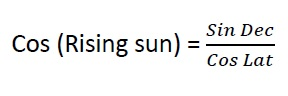Rising Az = E 34.5˚S
T Az          = 124.5˚ (T)
C Az          = 110.0˚  (C)
Error         = 14.5˚ E
Variation  = 10˚ E
Deviation = 4.5˚E

1. ###### Sept 23rd 2008, the sun rose bearing 094˚(C) in DR 00˚ 00’ 120˚ 27’W when chron showed 01h 54m. If chron error was 03m 12s FAST and variation was 2.7˚W, find the deviation for the ship’s head.

LMT Sunrise     = 23d   05h  49m  00s
LIT (W)                  = (+) 08h  01m  48s
GMT Sunrise      = 23d 13h  50m  48s

As we know that:
LMT of Sunrise or Sunset are taken from the nautical almanac and needs only be interpolated and taken in whole minutes of time. If the time ofobservation is given in the problem, then given time should be used for calculation. It is necessary to remove the ambiquity of chron time.

Dec sun  23d  13h             = S00˚20.7’
d(+01.0)                            =        + 00.8’
Dec 2d  06h  37m  00s    = S00˚  21.5’

We know that :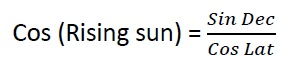###### NOTE:

If the latitude is 00  00’, the value of the amplitude is equal to the value of the declination. Naming of the amplitude is done as usual.

Rising Az = E00.4˚S
T Az          = 090.4˚ (T)
C Az          = 094.0˚  (C)
Error         = 3.6˚ W
Variation  =2.7˚ W
Deviation = 0.9˚W